# can someone please help me answer these, for some reason theh are both wrong and i cant get them right, will rate y...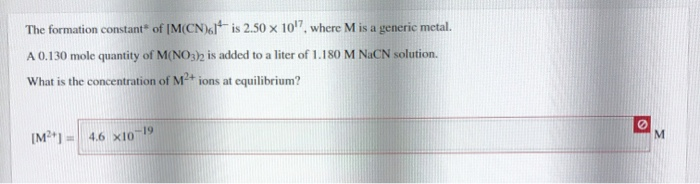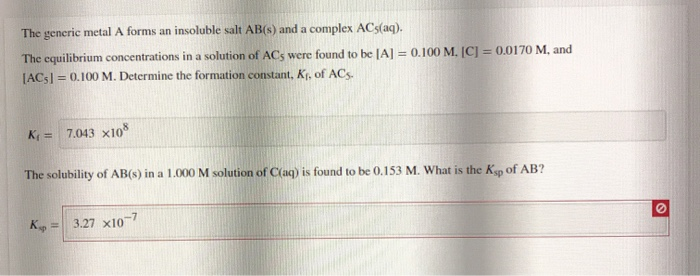can someone please help me answer these, for some reason theh are both wrong and i cant get them right, will rate you good if you can help get both correct answers! thanks
The formation constant of M(CN)61 is 2.50 x 10'7, where M is a generic metal. A 0.130 mole quantity of M(NO3) is added to a liter of 1.180 M NaCN solution. What is the concentration of M2+ ions at equilibrium? [M ] = 4.6 x10-19
The generic metal A forms an insoluble salt AB(s) and a complex ACs(aq). The equilibrium concentrations in a solution of ACs were found to be [A] = 0.100 M. [C] = 0.0170 M, and [ACs) = 0.100 M. Determine the formation constant, Ki, of ACs. Ki= 7.043 x108 The solubility of AB(s) in a 1.000 M solution of C(aq) is found to be 0.153 M. What is the Ksp of AB? Kp = 3.27 x10-7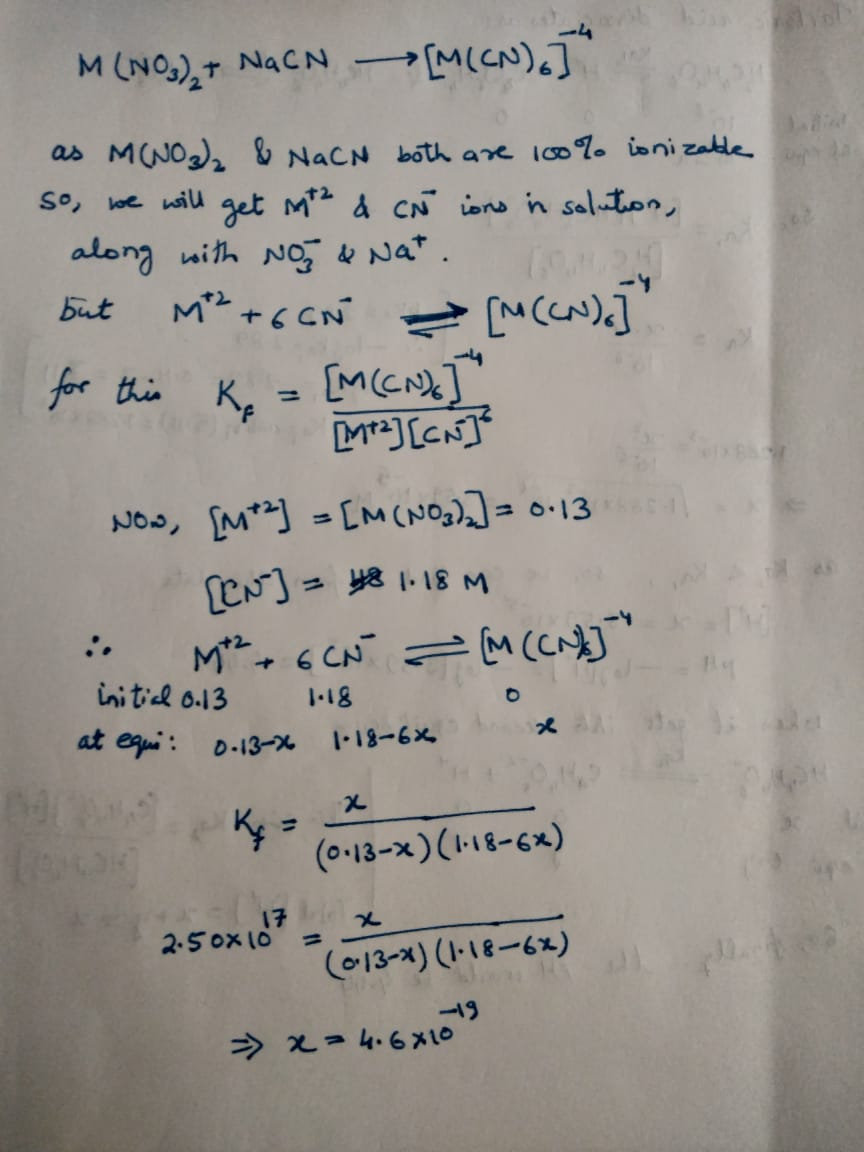#### Earn Coin

Coins can be redeemed for fabulous gifts.

Similar Homework Help Questions
• ### Question 4 of 5 > The generic metal A forms an insoluble salt AB(s) and a complex ACs(aq). The equilibrium conce...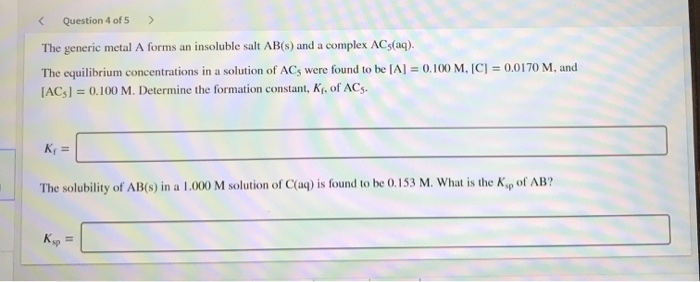Question 4 of 5 > The generic metal A forms an insoluble salt AB(s) and a complex ACs(aq). The equilibrium concentrations in a solution of ACs were found to be [A] = 0.100 M, [C] = 0.0170 M, and [ACs) = 0.100 M. Determine the formation constant, K. of ACs. The solubility of AB(s) in a 1.000 M solution of (aq) is found to be 0.153 M. What is the ke of AB? Ке

• ### Find Ksp The generic metal A forms an insoluble salt AB(s) and a complex ACs(aq). The...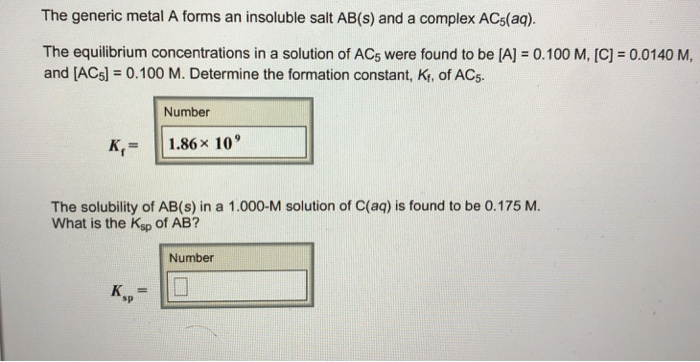Find Ksp The generic metal A forms an insoluble salt AB(s) and a complex ACs(aq). The equilibrium concentrations in a solution of ACs were found to be (A) = 0.100 M, [C] = 0.0140 M, and [ACs) = 0.100 M. Determine the formation constant, K, of AC5. Number K,= 1.86 x 10 The solubility of AB(s) in a 1.000-M solution of C(aq) is found to be 0.175 M. What is the Ksp of AB? Number

• ### The generic metal A forms an insoluble salt AB(s) and a complex AC5(aq). The equilibrium concentrations in a solution of...

The generic metal A forms an insoluble salt AB(s) and a complex AC5(aq). The equilibrium concentrations in a solution of AC5 were found to be [A]=0.100 M[A]=0.100 M, [C]=0.0260 M[C]=0.0260 M, and [AC5]=0.100 M[AC5]=0.100 M. Determine the formation constant, Kf, of AC5. ?f= The solubility of AB(s) in a 1.000 M solution of C(aq) is found to be 0.1310.131 M. What is the Ksp of AB? ?sp=

• ### The generic metal A forms an insoluble salt AB(s) and a complex AC5(aq). The equilibrium concentrations...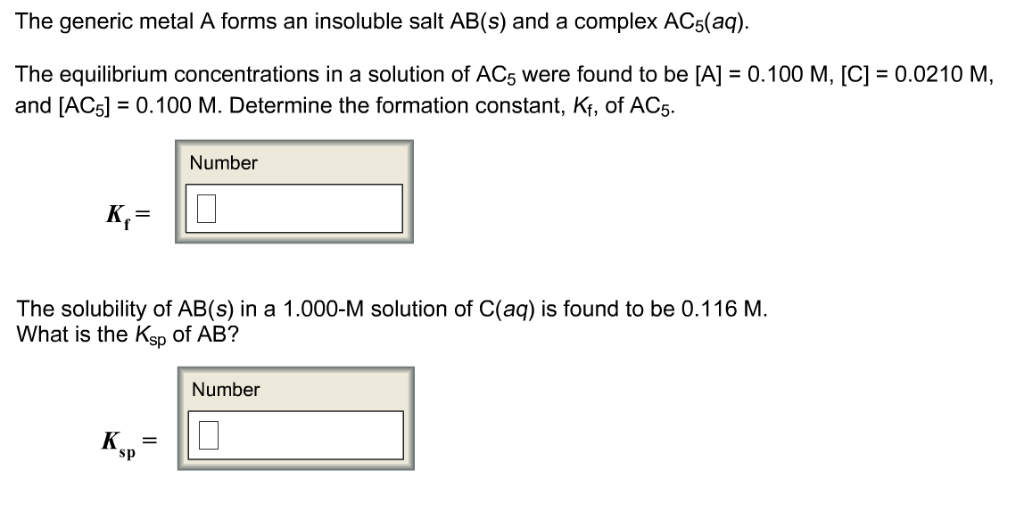The generic metal A forms an insoluble salt AB(s) and a complex AC5(aq). The equilibrium concentrations in a solution of AC5 were found to be [A] 0.100 M, [C] 0.0210 M, and [ACSl0.100 M. Determine the formation constant, K, of AC5 Number The solubility of AB(s) in a 1.000-M solution of C(aq) is found to be 0.116 M. What is the Ksp of AB? Number sp

• ### please help i cant figure them out Consider the insoluble compound nickel(II) carbonate, NiCO3. The nickel...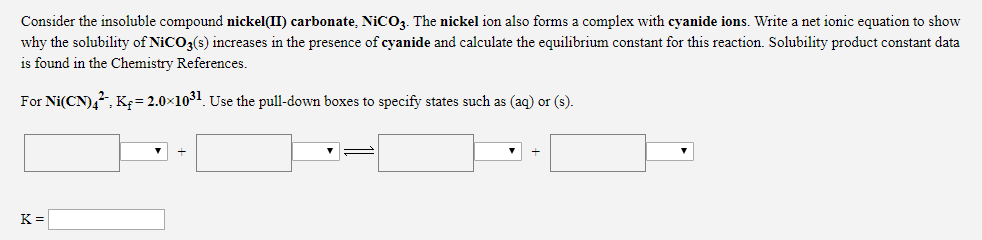please help i cant figure them out Consider the insoluble compound nickel(II) carbonate, NiCO3. The nickel ion also forms a complex with cyanide ions. Write a net ionic equation to show why the solubility of NiCO3(s) increases in the presence of cyanide and calculate the equilibrium constant for this reaction. Solubility product constant data is found in the Chemistry References. For Ni(CN)4?: K = 2.0 1031. Use the pull-down boxes to specify states such as (aq) or (s). A solution...

• ### i need help with this lab please. can i get someone to answer the entire thing....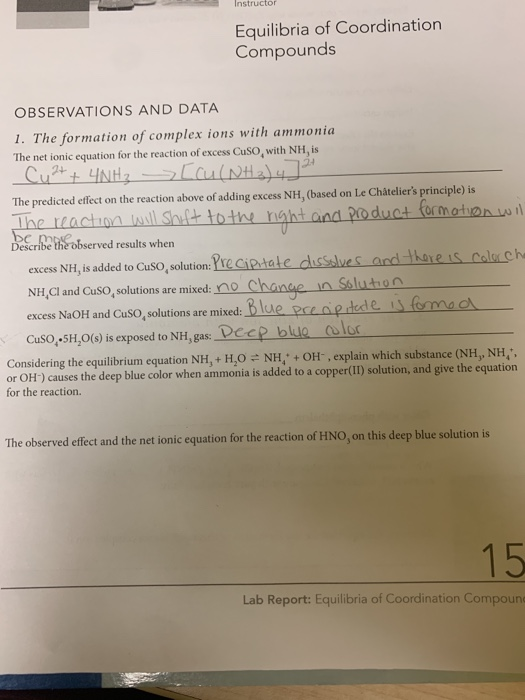i need help with this lab please. can i get someone to answer the entire thing. thank you Instructor Equilibria of Coordination Compounds OBSERVATIONS AND DATA 1. The formation of complex ions with ammonia The net ionic equation for the reaction of excess Cuso, with NH, is 24 Cu2+ + 4NH3 [cu (NH3)4] The predicted effect on the reaction above of adding excess NH, (based on Le Châtelier's principle) is The reaction will shift to the night and product formation...